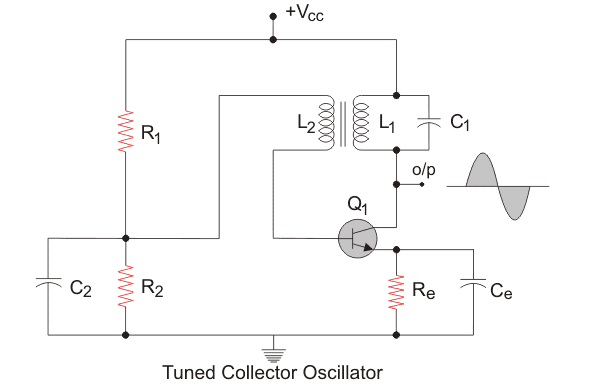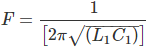# Tuned Collector Oscillator

Before we go into the topic of tuned collector oscillator, we must first understand what is an oscillator is and what it does. An oscillator is an electronic circuit that generates an oscillating or periodic signal, like a sine wave or a square wave. The main purpose of an oscillator is to convert a DC signal to an AC signal. Oscillators have numerous usages like in TV, clocks, radio, computers etc. Almost all electronic devices use some oscillators to generate an oscillating signal.

One of the simplest LC oscillators is the Tuned collector Oscillator. In the tuned collector Oscillator, we have a tank circuit consisting of a capacitor and an inductor and a transistor to amplify the signal. The tank circuit which is connected to the collector behaves like a simple resistive load at resonance and decides the oscillator frequency.

## Circuit Diagram Explanation of Tuned Collector OscillatorAbove is the circuit diagram of the tuned collector oscillator. As you can see, the transformer and the capacitor is connected to the collector side of the transistor. The oscillator here produces a sine wave.
R1 and R2 form the voltage divider bias for the transistor. Re refers to the emitter resistor and is there to provide thermal stability. Ce is used to bypass the amplified ac oscillations and is the emitter bypass capacitor. C2 is the bypass capacitor for resistor R2. The primary of the transformer, L1 along with capacitor C1 forms the tank circuit.

## Working of Tuned Collector Oscillator

Before we go into the working of the oscillator, let us just revise the fact that a transistor causes a phase shift of 180 degrees when it amplifies an input voltage. L1 and C1 form the tank circuit and it is from these two elements, we will get the oscillations. The transformer helps in giving a positive feedback(We will come back to this later) and the transistor amplifies the output. With that established, let us now proceed to understand the working of the circuit.

When the power supply is switched on, the capacitor C1 starts charging. When it is fully charged, it starts to discharge through the inductor L1. The energy stored in the capacitor in the form of electrostatic energy gets converted to electromagnetic energy and gets stored in the inductor L1. Once the capacitor discharges completely, the inductor starts charging the capacitor again. This is because inductors do not the current through them change quickly and hence it will change the polarity across itself and keep the current flowing in the same direction. The capacitor starts charging again and the cycle continues in this manner. The polarity across the inductor and capacitor changes periodically and hence we get an oscillating signal as the output.

The Coil L2 gets charged through electromagnetic induction and feeds this to the transistor. The transistors amplify the signal, which is taken as the output. Part of the output is fed back to the system in what is known as positive feedback.
Positive feedback is the feedback which is in phase with the input. The transformer introduces a phase shift of 180 degrees and the transistor also introduces a phase shift of 180 degrees too. So in total, we get a 360-degree phase shift and this is fed back to the tank circuit. Positive feedback is necessary for sustained oscillations.
The frequency of oscillation depends on the value of the inductor and capacitor used in the tank circuit and is given by:Where,
F = Frequency of the oscillation.
L1 = value of the inductance of primary of the transformer L1.
C1 = value of capacitance of capacitor C1.

Want To Learn Faster? 🎓
Get electrical articles delivered to your inbox every week.
No credit card required—it’s 100% free.